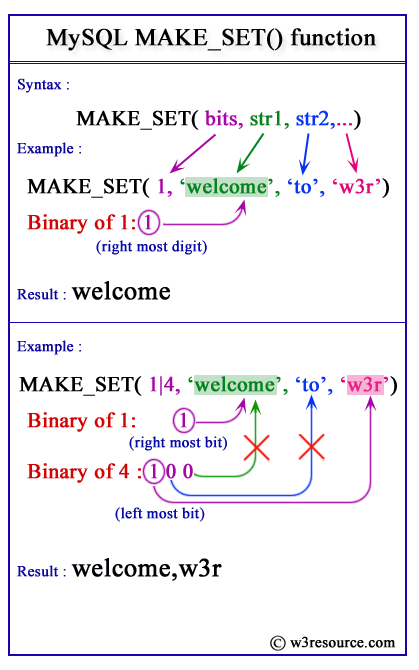# MySQL MAKE_SET() function

## MAKE_SET() function

MySQL MAKE_SET() returns a set value (a string containing substrings separated by “,” characters) consisting of the strings that have the corresponding bit in the first argument.

This function is useful in -

• String set creation: It allows us to create a string set representation based on a set of bit values.
• Bit value representation: MAKE_SET() can be used to convert bit values into a more readable and manageable string format.

Syntax:

```MAKE_SET (bits, str1, str2,….)
```

Arguments:

Name Description
bits An expression.
str1, str2,…. A list of strings.

MySQL Version: 8.0

Pictorial Presentation:Example -1: MySQL MAKE_SET() function

Code:

``````SELECT MAKE_SET(1,'a','b','c');
```
```

Explanation:

If the first argument (1) is converted to binary, it returns 1. So for the rightmost bit, the function returns 'a'. Since no other bits (obtained from the first argument) are available, the function does not add anything with 'a'.So the final output is 'a' .

Output:

```mysql> SELECT MAKE_SET(1,'a','b','c');
+-------------------------+
| MAKE_SET(1,'a','b','c') |
+-------------------------+
| a                       |
+-------------------------+
1 row in set (0.00 sec)
```

Example -2 : MySQL MAKE_SET() function

Code:

``````SELECT MAKE_SET(1 | 4,'hello','nice','world');
```
```

Explanation:

If the first argument (1 | 4) is converted to binary, it returns 1 or 100. For 1, the rightmost bit is 1, so the function returns 'hello'. For 100, the rightmost bit is 0, so the function returns nothing for the rightmost bit (0). But the function returns 'world' for the leftmost bit (1). So, the final output is hello,world.

Output:

```mysql> SELECT MAKE_SET(1 | 4,'hello','nice','world');
+----------------------------------------+
| MAKE_SET(1 | 4,'hello','nice','world') |
+----------------------------------------+
| hello,world                            |
+----------------------------------------+
1 row in set (0.00 sec)
```

Using the result with INSERT or UPDATE

Code:

``````CREATE TABLE colors (
id INT AUTO_INCREMENT PRIMARY KEY,
color_set SET('Red', 'Green', 'Blue', 'Yellow', 'Purple')
);

INSERT INTO colors (color_set) VALUES
(MAKE_SET(3, 'Red', 'Green', 'Blue')),
(MAKE_SET(9, 'Red', 'Yellow', 'Purple'));
```
```

Retrieving data from the SET column:

``````SELECT id, color_set FROM colors;
```
```

Output:

```id|color_set|
--+---------+
1|Red,Green|
2|Red      |
```

Video Presentation:

All String Functions (Slides presentation)

Previous: LTRIM
Next: MID

﻿